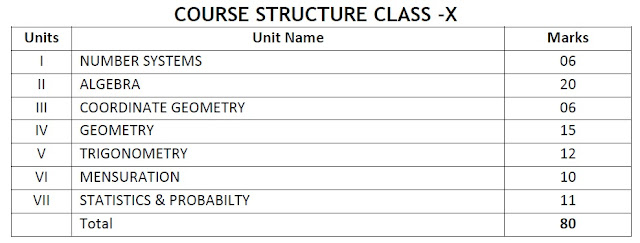## CLASS - X

CBSE BOARD EXAM 2018

VYAKHYAEDU APP - WATCH NOW

BEST APPS - WATCH NOW
BEST APP  - WATCH NOW

VIDEOS FOR CLASS X BOARD EXAM 2018 ( WATCH NOW )
•  watch Full Playlist of Class X WATCH NOW
• MATHS IMP. CHAPTERS - WATCH NOW
• Important Tips How to prepare to score High marks in CBSE class 10th or 12th Board Exam 2018

Mathematics (041) Class X sample question paper with important question & solution for Cbse board exam 2018 -- click here

## Other posts you might be interested in:

Mathematics (041) Class X sample question paper with important question & solution for Cbse board exam 2018 -- click hereMathematics (041) Class X sample question paper with important question & solution for Cbse board exam 2018 -- click here

Time allowed: 3 Hrs
Maximum Marks: 80

General Instructions:

(i) All questions are compulsory.

(ii) The question paper consists of 30 questions divided into four sections A, B, C and D.

(iii)Section A contains 6 questions of 1 mark each. Section B contains 6 questions of 2 marks each. Section C contains 10 questions of 3 marks each. Section D contains 8 questions of 4 marks each.

(iv) There is no overall choice. However, an internal choice has been provided in four
questions of 3 marks each and three questions of 4 marks each. You have to attempt only
one of the alternatives in all such questions.

(v) Use of calculators is not permitted.

Mathematics (041) Class X sample question paper with important question & solution for Cbse board exam 2018 -- click here

## UNIT I: NUMBER SYSTEMS

1. REAL NUMBER (15) Periods

## UNIT II: ALGEBRA

2. POLYNOMIALS (7) Periods

#### Zeros of a polynomial. Relationship between zeros and coefficients of quadratic polynomials. Statement and simple problems on division algorithm for polynomials with real coefficients.

3. PAIR OF LINEAR EQUATIONS IN TWO VARIABLES (15) Periods

#### Standard form of a quadratic equation ax2 + bx + c = 0, (a ≠ 0). Solutions of quadratic equations (only real roots) by factorization, by completing the square and by using quadratic formula. Relationship between discriminant and nature of roots.Situational problems based on quadratic equations related to day to day activities to be incorporated.

5. ARITHMETIC PROGRESSIONS (8) Periods

## UNIT III: COORDINATE GEOMETRY ch -7

1. LINES (In two-dimensions) (14) Periods

## UNIT IV: GEOMETRY ch- 6,10,11

6. TRIANGLES (15) Periods

#### Definitions, examples, counter examples of similar triangles.1. (Prove) If a line is drawn parallel to one side of a triangle to intersect the other two sides in distinct points, the other two sides are divided in the same ratio.2. (Motivate) If a line divides two sides of a triangle in the same ratio, the line is parallel to the third side.3. (Motivate) If in two triangles, the corresponding angles are equal, their corresponding sides are proportional and the triangles are similar.4. (Motivate) If the corresponding sides of two triangles are proportional, their corresponding angles are equal and the two triangles are similar.5. (Motivate) If one angle of a triangle is equal to one angle of another triangle and the sides including these angles are proportional, the two triangles are similar.6. (Motivate) If a perpendicular is drawn from the vertex of the right angle of a right triangle to the hypotenuse, the triangles on each side of the perpendicular are similar to the whole triangle and to each other.7. (Prove) The ratio of the areas of two similar triangles is equal to the ratio of the squares of their corresponding sides.8. (Prove) In a right triangle, the square on the hypotenuse is equal to the sum of the squares on the other two sides.9. (Prove) In a triangle, if the square on one side is equal to sum of the squares on the other two sides, the angles opposite to the first side is a right angle.

10. CIRCLES (8) Periods

#### Tangent to a circle at, point of contact1. (Prove) The tangent at any point of a circle is perpendicular to the radius through the point of contact.2. (Prove) The lengths of tangents drawn from an external point to a circle are equal.

11. CONSTRUCTIONS (8) Periods

## UNIT V: TRIGONOMETRY ch- 8 & 9

8. INTRODUCTION TO TRIGONOMETRY (10) Periods

#### Trigonometric ratios of an acute angle of a right-angled triangle. Proof of their existence (well defined); motivate the ratios whichever are defined at 0o and 90o. Values (with proofs) of the trigonometric ratios of 300, 450 and 600. Relationships between the ratios.

TRIGONOMETRIC IDENTITIES (15) Periods

## UNIT VI: MENSURATION

12. AREAS RELATED TO CIRCLES (12) Periods

#### Motivate the area of a circle; area of sectors and segments of a circle. Problems based on areas and perimeter / circumference of the above said plane figures. (In calculating area of segment of a circle, problems should be restricted to central angle of 60°, 90° and 120° only. Plane figures involving triangles, simple quadrilaterals and circle should be taken.)

13. SURFACE AREAS AND VOLUMES (12) Periods

## UNIT VII: STATISTICS AND PROBABILITY

14.STATISTICS (18) Periods

#### Mean, median and mode of grouped data (bimodal situation to be avoided).Cumulative frequency graph.

15. PROBABILITY (10) Periods

## PRESCRIBED BOOKS

Mathematics - Textbook for class X - NCERT Publication
Mathematics exemplar problems for class X, NCERT publication.
Guidelines for Mathematics Laboratory in Schools, class X - CBSE Publication